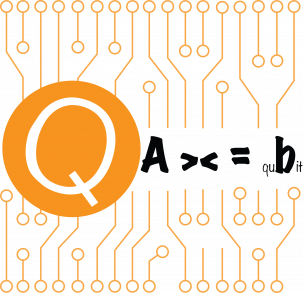Also see the Arxiv version (less frequently updated) arXiv:2201.08309

This is a set of lecture notes used in a graduate topic class in applied mathematics called ``Quantum Algorithms for Scientific Computation'' at the Department of Mathematics, UC Berkeley during the fall semester of 2021. These lecture notes focus only on quantum algorithms closely related to scientific computation, and in particular, matrix computation. The main purpose of the lecture notes is to introduce quantum phase estimation (QPE) and ``post-QPE'' methods such as block encoding, quantum signal processing, and quantum singular value transformation, and to demonstrate their applications in solving eigenvalue problems, linear systems of equations, and differential equations. The intended audience is the broad computational science and engineering (CSE) community interested in using fault-tolerant quantum computers to solve challenging scientific computing problems.

Please keep in mind that these are rough lecture notes and are not meant to be a comprehensive treatment of the subject. The notes are being continuously updated. For errors / comments / suggestions / general thoughts on the lectures notes, please send me an email: linlin at math dot berkeley dot edu.Contents

I. Preliminaries of quantum computation
1. Postulates of quantum mechanics
2. Density operator
3. Quantum circuit
4. Copy operation and no-cloning theorem
5. Measurement
6. Linear error growth and Duhamel’s principle
7. Universal gate sets and reversible computation
8. Fixed point number representation and classical arithmetic operations
9. Fault tolerant computation
10. Complexity of quantum algorithms
II. Grover’s algorithm
1. The first quantum algorithm: Deutsch’s algorithm
2. Unstructured search problem
3. Amplitude amplification
4. Lower bound of query complexity
III. Quantum phase estimation
2. Quantum phase estimation (Kitaev’s method)
3. Quantum Fourier transform
4. Quantum phase estimation using quantum Fourier transform
5. Analysis of quantum phase estimation
IV. Applications of quantum phase estimation
1. Ground state energy estimation
2. Amplitude estimation
3. HHL algorithm for solving linear systems of equations
4. Example: Solve Poisson’s equation
5. Solve linear differential equations
6. Example: Solve the heat equation
V. Trotter based Hamiltonian simulation
1. Trotter splitting
2. Commutator type error bound
VI. Block encoding
1. Query model for matrix entries
2. Block encoding
3. Block encoding of s-sparse matrices
4. Hermitian block encoding
5. Query models for general sparse matrices
VII. Matrix functions of Hermitian matrices
1. Qubitization of Hermitian matrices with Hermitian block encoding
2. Application: Szegedy’s quantum walk
3. Linear combination of unitaries
4. Qubitization of Hermitian matrices with general block encoding
5. Quantum eigenvalue transformation
6. Quantum signal processing
7. Application: Time-independent Hamiltonian simulation
8. Application: Ground state preparation
VIII. Quantum singular value transformation
1. Generalized matrix functions
2. Qubitization of general matrices
3. Quantum singular value transformation
4. Application: Solve linear systems of equations
5. Quantum singular value transformation with basis transformation
6. Application: Grover’s search revisited, and fixed-point amplitude amplification